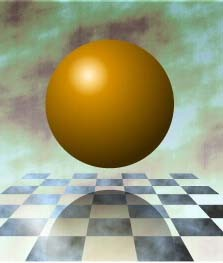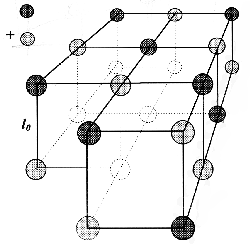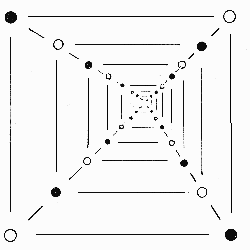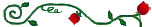EPOLA: A New Approach to the Fine Structure of Matter and Space Menahem Simhony Retired Associate Professor, Physics Section 5, The Hebrew University, Jerusalem, Israel Article 1

WHY ARE THERE: INERTIA, GRAVITY, QUANTIZATION,
THE OTHER "UNEXPLAINABLE" FACTS OF NATURE,
AND WHAT IS THE REAL MEANING OF E=mc2

1. Introduction
The White House Statement, Science in the National Interest, issued 1994, calls to improve science education and increase public understanding of science. In the achievement of these two goals, the natural science of physics (physis means nature in Greek) should play a leading role, because physics deals with the simplest phenomena of Nature. The real understanding of physical phenomena is therefore crucial for the deep understanding of other natural sciences, and essential for the improvement of science education.The status of understanding physics was best assessed by Richard Feynman, probably the greatest theorist of the 70s and 80s, who wrote: "I think I can safely say that nobody understands quantum mechanics". This "nobody understands" equally relates to relativity as to all "new physics". People who think or say that they understand physics, may actually understand the mathematics employed to solve physical problems. They may also consider as understanding the ability to quote an axiom, postulate or an unexplained saying by an authority. Such a sense of understanding is right in mathematics and in scholastic philosophies, not in natural sciences.

Physics is unable to explain the most basic questions, like WHY is there inertia, gravitation, and HOW does space carry, transfer, and propagate gravitational forces, and the tremendous forces and energies of electro-magnetic interactions and radiation. Modern physics gets rid of such questions by proudly declaring the absence of physical explanations and understanding, thus implying the divinity of its postulates and of its mathematically derived findings. Anyone asking the WHY and HOW questions risks to be proclaimed a fool, as were the unbelievers in H.C. Andersen's tale about the new clothes of the emperor.

2. Consequences of Mathematical Dealing with Physical Phenomena
Unlike other natural sciences, physics is unable to explain physical facts of everyday life; e.g., asked why is it so hard to stop a speeding car at a sudden obstacle, a physicist will say: "because of inertia" or at best, "because of Newton's Laws of Motion". But the physical reasons, causes, and mechanisms of inertia were never explained, neither were Newton's laws of motion. These basic laws were just postulated, i.e., introduced like axioms in mathematics, without answering WHY questions.

The physicist, let alone the laymen, does not realize that naming or quoting an unexplained phenomenon or postulate is just diagnosing, at best, not explaining. He will recite the law, write the formulae, show how to calculate the force needed to stop the car, and this is to him the best and only "explanation". If you continue the WHY quest, you may be pronounced a nuisance or fool.

Mathematics can provide calculated solutions to thousands of physical problems. The solutions may also illustrate physical facts, but cannot prove or explain them. Contrary to popular beliefs, mathematical proofs can only prove that the mathematical approach is applicable, but cannot prove that the physical picture or model behind it is right. Influenced by mathematical approaches, and being paid, published, promoted for using and developing them, physicists are not interested to disclose the physical causes of phenomena. They are often reluctant to do so, as if it meant dealing with some kind of dirt, as opposed to the purity of mathematics and "the beauty in one's formula" (P.A.M. Dirac).

The unanswered "why" questions make students either leave physics or become sufficiently brainwashed to believe that there is no physical understanding but postulated laws, axiomatic principles, and mathematical formulae, for calculations that satisfy the immediate needs of people working in physics and around it.

3. Wrong Concepts and Misinterpretations of ExperimentsSince March 1973, this author has been arguing that the inability to understand physics is due to our stubborn sticking to the concepts that space without bodies (the "vacuum" space) is absolutely empty, and that bodies and ambient media are continuous. A science, based on a physically unfeasible model, adjusts its mathematical and scholastic methods, logic and theories to fit measured data. So it was for two millennia, when the stars, the sun and the planets were considered to circle around earth (geo) in the physically unfeasible geo-centric model. So it is now in physics, which is based on the physically unfeasible and unachievable absolutely empty `vacuum' space. A science, based on erroneous ideas, twists experimental results, definitions and laws, to make them "prove" these ideas. When the ideas are replaced by contradicting ones, then the same results "do just as well or better in proving the new ones" (Max von Laue).

For example, the Michelson-Morley Experiments (1887) proved that the motion of an atomic body does not cause winds in the carrier of light, the "ether". Motion of a continuous body in the continuous ether must cause winds in it. Michelson thus decided that "no ether winds" means "no ether", and no material carrier of light at all. This is twisted logic; the straight one is, that if the light-carrying space is not continuous but consists of discrete particles, located far apart from one another, then the motion in it of bodies, consisting of similarly discrete particles, would not be able to cause winds in it.

Indeed, 24 years later, Rutherford's Experiments have proven (in 1911), that all atoms, ions, molecules, and atomic bodies, including our own, as well as earth, the planets, the sun, consist of nothing else but nuclear particles, atomic nuclei and electrons.  The distances between neighboring nuclear particles in the bodies are a hundred thousand times larger than the sizes of these particles.  Hence, the discrete atomic bodies can move in a discrete space without causing winds in it. Thus Rutherford's experiments not only prove that atomic bodies are not continuous but discrete (and very much so!).  They also allow us to say that the absence of "ether winds" proves that a light-carrying medium, if any, must be discrete.

4. Anderson's Experiments and the E=mc2 Formula
The Anderson Experiments of 1932 have proven that when a cosmic gamma-ray quantum, of energy at least 1.02 million electron volt (1.02 MeV), is absorbed in any point of space, then a free electron and a free positron appear in this point. When such two particles approach each other slowly in any point of space, they may disappear to our apparatus, with the emission out of this point of two (at least) radiation quanta. The energy sum of the emitted quanta is then 1.02 MeV.

Analogous processes occur in ionic crystals; e.g., when ultraviolet radiation of at least 8 eV photon energy is absorbed in a sodium chloride (table salt) crystal, then pairs of free sodium and chlorine ions appear in the crystal, one per each absorbed photon. The freed ions can be detected due to some electrical conductivity and opaqueness they cause in the crystal. When such two ions are captured into bonds in the crystal, the 8 eV energy is emitted in the form of at least two photons. The captured ions disappear to our apparatuses, which are unable to detect them.Figure 1.  The Sodium-Chloride-Type Cubic Lattice. Light circles represent positive charges (of sodium ions in the table salt crystal, of positrons in the epola). Dark circles represent negative charges (of chlorine ions in the crystal, of electrons in the epola). In the scale of the edges l0 of the cubes, the radii of the circles are 3 to 5 times too small to depict the radii of the ions, and 6 times too large to depict the radii of epola electrons and positrons.

The freeing and capture (binding) processes fit the E=mv2 formula, where E=8 eV is the binding energy of the ion pair, m is the mass of the pair, and v is the average velocity of elastic waves in the crystal. The E=mv2 formula follows from the formula v2=E/m for the average velocity v of sound in each of the 17 sodium-chloride-type ionic crystals. Substitution of data yields v=3.6 km/s (3.6 kilometers per second), which is the experimental value of the average sound velocity in the sodium chloride crystal.

We thus interpret the results of Anderson’s Experiments as showing that space contains bound electrons and positrons, and that the binding energy of the pair of particles in space is 1.02 MeV. Absorption of a quantum of this energy frees the pair out of bonds in space, making the free particles appear to the detecting apparatus. When a pair of such particles is captured into bonds in space, then this binding energy is emitted in at least two quanta of radiation. Particles captured into bonds in space disappear to our apparatuses, which are unable to detect them.

By analogy with the sodium chloride crystal, the velocity c of light in space should be c2=E/m. Indeed, substitution of 1.02 MeV for E, and of the known value of the mass m of the electron-positron pair, yields for the velocity of light in space the experimental value of c=300,000 km/s. This is one of the numerous strong indications of not only the fact that space contains bound electrons and positrons, but also that these particles are ordered in space to form a lattice, similar to the lattice of the sodium chloride crystal.

The formula for the velocity of light, c2=E/m, can easily be transformed into E=mc2, which reads, that the energy E, absorbed to free a mass m of electrons and positrons (or other nuclear particles) out of their bonds in space, is equal to the mass m of the freed (appeared) particles, multiplied by the squared velocity of light in space; or that the energy E, emitted when a mass m of nuclear particles "falls" into bonds in space, is equal to the mass m of these disappeared particles, multiplied by the squared velocity of light in space.

5. The Mass-Energy Equivalence Deception
In 1905, Einstein derived the E=mc2 formula for the radiation energy of an electron moving in the electro-magnetic field. Then he stated that the formula is valid for bodies of atomic matter. But nuclear matter is 14 orders of magnitude (hundred thousand billion times) denser than atomic matter. Moreover, the electric charge per unit mass of the electron is 42,000 times higher than in the sodium ion, which is, in turn, trillions of times higher than in the strongest static charges of atomic bodies. Thus, what is right for a nuclear particle does not become right for an atomic body, especially not by just saying so.

Einstein also equated the electromagnetic radiation energy, for which he derived the E=mc2 formula, to any other energy, by simply declaring that: "The fact that the energy withdrawn from the body becomes energy of radiation evidently makes no difference, so that, The mass of a body is a measure of its energy content; if the energy changes by E, the mass changes in the same sense by E/c2." But it evidently makes a difference; a great one.

Kinds of energy are not equal, one kind may do what no other can. Some kinds are just "more equal"; thus, only a small part of the thermal energy can be converted into mechanical energy. The energy of electric currents is most versatile, but only a part of it can be converted into radiation energy, the "most equal" kind. The law of Energy Conservation states that when an energy is converted into another kind, then the converted amounts of energy are equal. Different kinds of energy are not equal and cannot be replaced by one another.

Moreover, mathematical equations contain amounts only and can thus equate amounts only. They cannot equate what they don’t have: kinds of energy, of matter, force, other physical magnitudes, or people. All the more so, equations cannot equate or replace energy by mass or mass by energy. Therefore, Einstein's generalization of the E=mc2 formula and its presentation as a "mass-energy equivalence" is improper, to say the least.

Until 1932, this interpretation of the E=mc2 formula had little use, and no experiment to "prove" it. Then the Anderson experiments were interpreted as proving that the energy of 1.02 MeV is turning into, or creating an electron positron pair, and that the pair is turning or annihilating into the energy of 1.02 MeV. This interpretation is not only twisted, but also destroys the established physical definitions of mass, of energy, and of the Law of Mass Conservation "the most elaborately proven law of physics and chemistry". Moreover, never was there a single electron or a single positron "created" out of space, nor "annihilated" into space by any achieved energy, of tens of MeV, of hundreds, of thousands, not with the recently achieved energies up to a million MeV. Hence, 1.02 MeV cannot "create" an electron and a positron, if up to million times higher energies cannot "create" out of space either one of these particles.

6. The Proposed Electron Positron Lattice (EPOLA) Structure of Space
The almost undisturbed motion of alpha particles through atomic bodies, disclosed in the Rutherford Experiments, and the even lesser disturbance of atomic bodies to the motion of neutrons in them, means that atomic bodies can move just as undisturbed through collectives of these nuclear particles. This is so because the distances between the nuclear particles, constituting the atomic bodies, are up to a hundred thousand times larger than the sizes of these nuclear particles. Proportionally, a net built of a tenth of a millimeter thin nodes, ten meters apart from one another, could move undisturbed through plankton, and even through schools of sardines and herring. Consider now an electrically neutral collective of electrons and positrons, elastically bound to one another at inter-particle distances 50 times larger than their radii. This distance fits the medium size atomic nuclei. Now suppose that space around us represents such a network, or lattice of electrons and positrons, bound by 1.02 MeV per pair, a hundred thousand times stronger than strongest bound solids. Its particles would not be detected by our "regular" means, nor pumped out by any and all vacuum pumps.

 l0Figure 2.  Unit Cubes of the Epola.   The epola space in front of an approaching free nuclear particle  or atomic nucleus.

An atomic body, moving in this lattice, would "sweep" the nuclei and electrons of its atoms through the interparticle distances in the lattice, causing no winds or currents, only vibrations and waves. These waves appear as "waves of matter" or "de Broglie waves", introduced in quantum theory by L.V. de Broglie in 1924. Similarly, a net of thin cord and large eyelets does not cause currents, when moving in water, only wavelets around the nodes. Hence, the Rutherford and Anderson experiments lead also to a physical explanation of the "no ether winds" results of the Michelson-Morley experiments: The motion of an atomic body in the vacuum space makes no winds in it,
because this is not the motion of a dense continuous wall in a continuous ambient medium,
as we are used to think, but the motion of a highly "diluted", rare network of nuclear particles
through a lattice of nuclear particles, positioned far apart from one another.

The nuclear particles, constituting the atoms of the body, constantly interact with the epola particles.  When the atomic body rests, the interaction deforms the body and the lattice, resulting in gravitational, electric, and magnetic forces.  When the body moves, the interactions result additionally in the observed de Broglie waves in the epola space and cause inertia. The interactions also raise the observed "effective" mass of nuclear particles when their velocity approaches the speed of light.

7. The Physical Nature of Inertia
Epola units expand when entered by a nuclear particle, and contract when the particle leaves.  If the particle moves in the epola with a velocity v, much lower than the speed of light, then the epola units along the path of the particle vibrate with a frequency f, proportional to the velocity of the particle.   These vibrations create an electro-magnetic (epola) spacewave of de Broglie wavelength w. The spacewave propagates with the speed of light ("wave velocity" of quantum physics), well ahead of the particle, pre-forming the epola for its motion by creating a narrow wave-channel around its path. In this "accompanying spacewave" channel, the epola does not resist the uniform motion of the particle.

Why is there inertia?  There is inertia, because around the path of each nuclear particle of a moving body, there are quintillions of epola particles vibrating at a frequency proportional to the velocity of the particle. As long as the velocity of the particle is constant, the vibrational frequency and energy of these tremendous amounts of epola particles remain constant. Any change in the velocity of a nuclear particle requires a corresponding change in the vibrational frequency and energy of these particles.

Starting the motion of a body relative to the epola requires an energy supply to make the surrounding epola particles vibrate and create the accompanying spacewaves around each nuclear particle of the body. The need to provide this energy is said "due to inertia", to the inertial trend of the body to preserve its state of relative rest. The provided energy is then the kinetic energy of the body.

Stopping the motion requires outlets, able to absorb the energy of the accompanying waves of all nuclear particles of the moving body (friction in brakes, tires, runway; air resistance in raised ailerons, released parachute, etc.).  The need to provide such outlets is said "due to inertia", to the inertial trend of the body to keep its velocity constant.  Energy must be taken away from the system, consisting of the moving body and the spacewaves of all its nuclear particles.  This energy is considered the kinetic energy of the body.  Hence, inertia is as much a "belonging" or feature of particles of nuclear matter and of bodies consisting of such particles, as it is a property of the surrounding epola space.

Our explanation provides the ability to derive Newton’s laws of motion, the formulae for kinetic energy, momentum conservation, etc. This is an indication of the validity of the epola structure of space.  The epola model allows us also to understand the never explained physical mechanisms of gravitation,  of electro-magnetic interactions and propagation of electromagnetic radiation;  to prove the absurdity and physical baselessness of various big-bangs, interstellar travel, time machines, etc.; to explain the possible physical nature of black holes, the mechanisms of the creation of atomic matter by epola collapse, the recent (1997 Nobelist’s) discovery of the viscous "optical molasses" -like behavior of space at very low temperatures, and other physical facts and problems.

If you are interested to find out what the Epola model of space does and can do for the understanding of observed physical phenomena, I may recommend a close encounter with my book  Invitation to the Natural Physics of Matter, Space, and Radiation, World Scientific Publishing Co, 1994, (292 pages, ISBN 981-02-1649-1, can be ordered from Amazon.com  or Barnes & Noble).

All mathematical derivations can be found in my Paperback, The Electron-Positron Lattice Space, Cause of Relativity and Quantum Effects , Physics Section 5, The Hebrew University, Jerusalem 1990 (158 pages). The Paperback, as well as my popular Booklet, The Story of Matter and Space, 1999 (70 pages) can be ordered from Robi Guttman - guttmans@netvision.net.ilDr. M. Simhony, 33 Shoham Street, 34679 Haifa, Israel
Fax: 972 4 825 1681. E-mail: msimhony@hotmail.com

Top of Page   / Home Page   /  The Booklet  /  The Paperback   /  The Book  /  Publications   /  Affiliations

More Articles:

Updated:  12/2000

 Design and Promotion by High Brow Surfer Editor of Internet Site: Robi Guttman